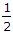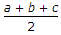# Civil Engineering - Surveying

### Exercise :: Surveying - Section 2

41.

Pick up the correct statement from the following :

 A. the power of a lens is the reciprocal of its focal length B. the unit of power of the lens is diopter C. the power of two or more thin lenses in contact is the power of the combination of the lenses D. all the above.

Explanation:

No answer description available for this question. Let us discuss.

42.

The area of a plane triangle ABC, having its base AC and perpendicular height h, is

 A.bh B.ba sin C C.bc sin A D. S(S - a)(S - b)(S - c) where S isE. all the above

Explanation:

No answer description available for this question. Let us discuss.

43.

The vertical angle between longitudinal axis of a freely suspended magnetic needle and a horizontal line at its pivot, is known

 A. declination B. azimuth C. dip D. bearing.

Explanation:

No answer description available for this question. Let us discuss.

44.

A relatively fixed point of known elevation above datum, is called

 A. bench mark B. datum point C. reduced level D. reference point.

Explanation:

No answer description available for this question. Let us discuss.

45.

Cross hairs in surveying telescopes, are fitted

 A. in the objective glass B. at the centre of the telescope C. at the optical centre of the eye piece D. in front of the eye piece.